# Using cyclomort: A tool for estimating seasonal mortality patterns

#### 2020-08-12

The cyclomort package presents a selection of tools for fitting, exploring, and visualizing uni- or multi-modal periodic hazard functions to right-censored mortality data. Its development was motivated by the fairly common observation in wildlife populations that the risk of mortality is higher in certain times of year than others. The central function, fit_cyclomort, returns estimates of the timing, duration, and intensity of one or more “mortality seasons”. Other functions summarize, visualize, and perform some statistical tests comparing models. The underlying model and its implementation are described in detail in Gurarie et al. (in review), and users are encouraged to read the paper for a deeper understanding of the likelihood based estimation of the parameters. This vignette illustrates the basic use of the most important functions.

To load the package:

library(cyclomort)

## Underlying wrapped Cauchy hazard model

The underlying hazard model is based on a mixture of a modified wrapped Cauchy-like functions. Its simplest iteration is wc(), parameterized in terms of a mean mu, a concentration parameter rho which ranges from 0 to 1, and a periodicity tau. Its mean value is 1. In the examples below, there is a single mortality season that peaks on day 100 on a period of 365 days (i.e. April 10 in a calendar year):

curve(wc(x, mu = 100, rho = .7, tau = 365), xlim = c(0,365), n = 1e4, ylab = "hazard", xlab = "time")
curve(wc(x, mu = 100, rho = .5, tau = 365), add = TRUE, col = 2)
curve(wc(x, mu = 100, rho = .3, tau = 365), add = TRUE, col = 3)
legend("topright", col = 1:3, legend = c(.7,.5,.3), title = "rho", lty = 1)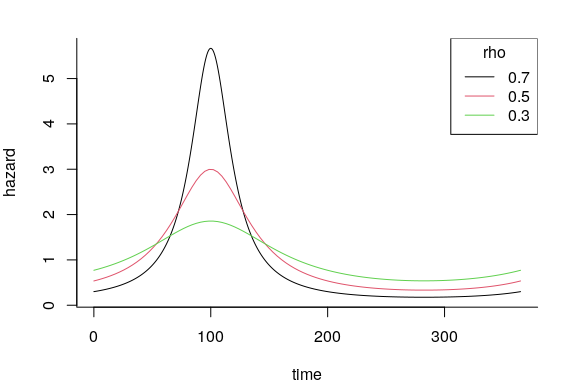The mixture version mwc() takes vectors of the means and concentration parameters, as well as a vector mean hazard values gammas. The sum of gammas is the overall mean hazard. Several examples (on a periodicity of 1, with an overall mean hazard 1) are illustrated below:

mus <-  c(.3,.5,.9)
rhos <-  c(.5,.9,.7)
gammas <- c(.6,.1,.3)
curve(mwc(x, mus = mus, rhos = rhos, gammas = 1, tau = 1),
xlim = c(0,1), ylab = "hazard", xlab = "time")
curve(mwc(x, mus = mus[1:2], rhos = rhos[1:2], gammas = gammas[1:2]/sum(gammas[1:2]), tau = 1),
add = TRUE, col = 2)
curve(mwc(x, mus = mus, rhos = rhos, gammas = gammas, tau = 1),
add = TRUE, col = 3)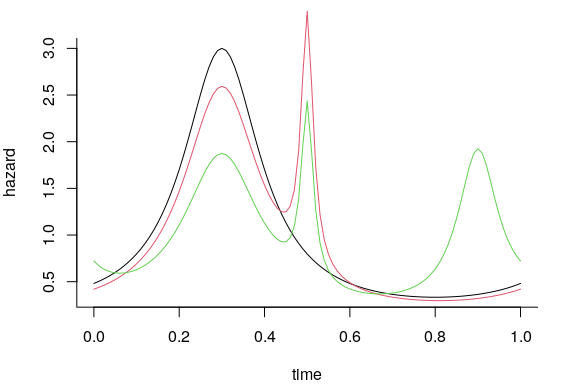Note that in the three season model above, the second peak is quite pronounced (i.e. has the highest instantaneous hazard), but it accounts for only 1/10 of the total hazard since it has such a short duration.

Additionally there are functions for the integrals of these functions : iwc() and imwc() respectively. It is important to remember that these functions are not probability distributions (or, respectively, cumulative probability distributions) but positive recurring functions with an infinite support, the long-term mean of which is the sum of gamma vector.

This set of functions are mainly internal and used for fitting, though they can be useful for visualizing the shape of an underlying hazard. In subsequent analyses and applications, the parameter rho is replaced with a “season duration” parameter, and an additional parameter of the mean overall hazard. In turn, gammas are converted to “weight” parameters for each component, with all weights from each component summing to 1.

## Simulating periodic mortality processes

The simulate_cycloSurv() function generates time-to-event data from an underlying periodic hazard process. Note that this function is parameterized via the (spelled-out) parameters: period, meanhazard, peaks, durations and weights. By default, this function plots the hazard, cumulative mortality, survival curve, and a histogram of simulated mortalities:

T.morts.sim <- simulate_cycloSurv(n = 300, period = 365, meanhazard = 1/365,
peaks = c(100, 250), durations = c(25, 40),
weights = c(0.6, 0.4), plotme = TRUE,
max.periods = 5)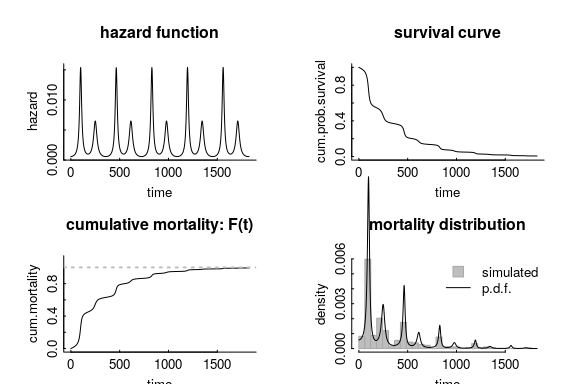The function returns an object of the cyclomort specific class cycloSurv, which is a superclass of the Surv data type used widely in survival analysis in R:

T.morts.sim[1:10]
>   (0,199.59784+] (0, 58.65910+] (0, 98.64865]  (0,515.16517]  (0,851.30130]
>   (0,443.91892]  (0,213.73874]  (0,111.43644]  (0,162.58759]  (0,228.35335]
class(T.morts.sim)
>  "cycloSurv" "Surv"

## Fitting a periodic hazard process

The fit_cyclomort() function estimates the parameters of the periodic hazard process. Note that we have to specify the numbers of seasons to fit.

T.morts.fit <- fit_cyclomort(T.morts.sim, n.seasons = 2)

The returned object is of class cmfit, which has summary, print and plot methods:

print(T.morts.fit)
> Multi-seasonal hazard function fit with 2 seasons with periodicity 365.
>
> $meanhazard > estimate CI.low CI.high se > 1 0.003057972 0.002804091 0.003311852 0.0001269402 > >$season 1
>   parameter season   estimate    CI.low     CI.high
> 1      peak      1 101.943297 99.066541 104.8200521
> 2  duration      1  25.042861 18.955380  32.7295655
> 3    weight      1   0.568094  0.460535   0.6756531
>
> $season 2 > parameter season estimate CI.low CI.high > 1 peak 2 242.475409 230.6946398 254.2561783 > 2 duration 2 57.826702 37.8705453 82.5525044 > 3 weight 2 0.431906 0.3054071 0.5584048 > > Log-likelihood: -104.502; AIC: 221.004 From this summary, we see that the true values are within the 95% confidence intervals of the estimates. The default plot: plot(T.morts.fit, breaks = 30) > Estimating 95% confidence intervals for the hazard function drawing 5000 samples from the parameter estimates.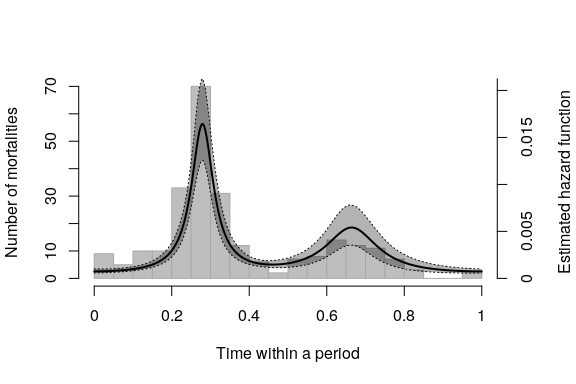By default, the function plots a histogram of the data as well. It can be useful to plot only the fit and the confidence interval, for example to compare the 2 season fit with a 1 season and null fit, while suppressing the histogram, for example: T.morts.1season.fit <- fit_cyclomort(T.morts.sim, n.seasons = 1) > Warning in fit_cyclomort(T.morts.sim, n.seasons = 1): Upper confidence limit for > weights manually coerced to 1 T.morts.null.fit <- fit_cyclomort(T.morts.sim, n.seasons = 0) plot(T.morts.fit, histogram = FALSE, monthlabs = TRUE) plot(T.morts.1season.fit, histogram = FALSE, add = TRUE, hazcolor = "red") plot(T.morts.null.fit, histogram = FALSE, add = TRUE, hazcolor = "blue")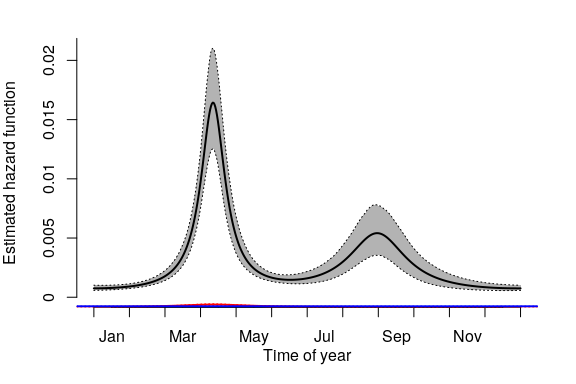The plot of the fitted objects includes confidence intervals around the predictions. These are obtained numerically by generating a hazard curve many (by default: 5000) times using parameter value sampled from the MLE estimates using the Hessian to generate a variance covariance matrix across the parameters. This procedure is performed with the predict method (predict.cmfit function), which returns a set of predictions for a given fit at a time or set of times with a specific parameter sets. Users can also call predict.cmfit to estimate the expected time to death for any individual at any given time. Note, this procedure takes a very (in fact, embarrassingly) long time even with relatively few samples (e.g. 100). We are working on this! ## timetoeventprediction <- predict(T.morts.fit, t = 1:365, type = "timetoevent", CI = TRUE, nreps = 100) The figure below illustrates the extent to which the expected time to an event depends on when an individual “enters” the process. with(timetoeventprediction, { plot(t, fit, type = "l", ylab = "Time to event", ylim = range(CI), lwd = 2) lines(t, CI[1,]) lines(t, CI[2,]) })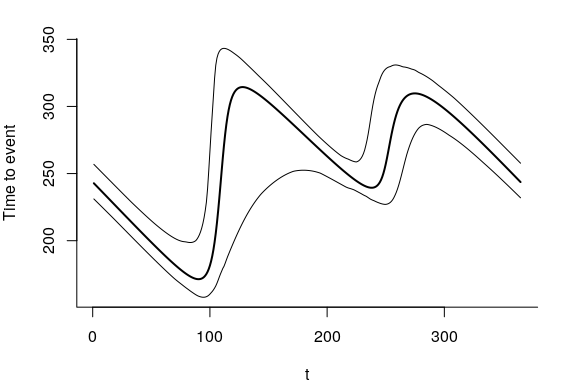## Identifying the number of mortality seasons within a system using select_seasons An important aspect of survival modelling is the ability to definitively characterize specific traits of a data set or population. Identifying the probable number of mortality seasons in a system is a powerful and useful claim that one can make using the select_seasons function. This function calls fit_cyclomort for a number of different seasons (ranging from the null model of 0 seasons to the max.season parameter), identifying the best model using Akaike Information Criterion (AIC). The select_seasons function returns a cmfitlist object which is equipped with unique print and summary functions. While cmfitlist objects are simply lists of cmfit objects (each element of the list being the cmfit object representing a different seasonal assumption), the unique summary.cmfitlist function allows for easier comparison of the different fits. T.select <- select_seasons(T.morts.sim, max.season = 4) > Fitting model with 0 seasons... > Fitting model with 1 seasons... > Warning in fit_cyclomort(x, n.seasons = n.seasons): Upper confidence limit for > weights manually coerced to 1 > Fitting model with 2 seasons... > Fitting model with 3 seasons... > Fitting model with 4 seasons... > Warning in sqrt(diag(solve(fits$hessian))): NaNs produced
> Warning in fit_cyclomort(x, n.seasons = n.seasons): Could not produce confidence intervals for mean hazard parameter. Interpret results with skepticism.
> Warning in fit_cyclomort(x, n.seasons = n.seasons): Could not produce accurate confidence intervals for weight parameter. Interpret results with skepticism.
> Warning in fit_cyclomort(x, n.seasons = n.seasons): Could not produce confidence intervals for peak parameter. Interpret results with skepticism.
> Warning in fit_cyclomort(x, n.seasons = n.seasons): Could not produce confidence intervals for duration parameter. Interpret results with skepticism.
>
> Delta AIC table of fitted models:
>  n.seasons    logLik d.f.      AIC       dAIC
>          0 -214.3846    1 430.7691 209.764713
>          1 -164.4372    3 334.8744 113.869963
>          2 -104.5022    6 221.0044   0.000000
>          3 -103.4557    9 224.9115   3.907071
>          4 -102.3909   12 228.7819   7.777458

As expected, the select_seasons function identifies the two season model as the one with the lowest AIC value. Note, the likelihood maximization algorithm had some difficulty obtaining the Hessian matrix for the overly complex 4 season model, but (in our experience) the inference for model selection is still generally stable.

## Simple factor analyses

The factorfit_cyclomort function allows users to test whether or not a categorical variable has an impact on seasonal mortality patterns, using R’s formula notation. Input data for this function can be structured as a data frame with a categorical variable and a Surv (or cycloSurv) object in columns. There is a simulated dataset in the package called seasonalsex that illustrates a hypothetical example where factorial analysis may be useful:

data(seasonalsex)
str(seasonalsex)
> 'data.frame': 200 obs. of  2 variables:
>  $sex : Factor w/ 2 levels "F","M": 2 2 2 2 2 2 2 2 2 2 ... >$ event: 'cycloSurv' num [1:200, 1:3] (0,6.134+] (0,1.822]  (0,5.347+] (0,1.121]  ...
>   ..- attr(*, "dimnames")=List of 2
>   .. ..$: NULL > .. ..$ : chr [1:3] "start" "stop" "status"
>   ..- attr(*, "type")= chr "counting"
>   ..- attr(*, "period")= num 1
>   ..- attr(*, "t0")= num 0

The implementation is straightforward:

Survival.factorfit <- factorfit_cyclomort(event ~ sex, data = seasonalsex, n.seasons = 1)
> Warning in fit_cyclomort(t, n.seasons = n.seasons, ...): Upper confidence limit
> for weights manually coerced to 1
> Warning in fit_cyclomort(t.subset, n.seasons = n.seasons, ...): Upper confidence
> limit for weights manually coerced to 1

> Warning in fit_cyclomort(t.subset, n.seasons = n.seasons, ...): Upper confidence
> limit for weights manually coerced to 1
summary(Survival.factorfit)
> Summary table comparing factorial seasonal survival model with 1 seasons.
>
> Formula: event ~ sex
>        logLike k    AIC   LRT p.value
> null -339.2927 3 684.59 44.15       0
> sex  -317.2170 6 646.43

The analyses reports a p-value based on a likelihood ratio test of a model that includes the factor against a null model that does not, as well as reporting AIC values. A plotting method provides a visual comparison of the fits.

plot(Survival.factorfit, ymax = 1.2)
> Estimating 95% confidence intervals for the hazard function drawing 5000 samples from the parameter estimates.
>
> Estimating 95% confidence intervals for the hazard function drawing 5000 samples from the parameter estimates.
>
> Estimating 95% confidence intervals for the hazard function drawing 5000 samples from the parameter estimates.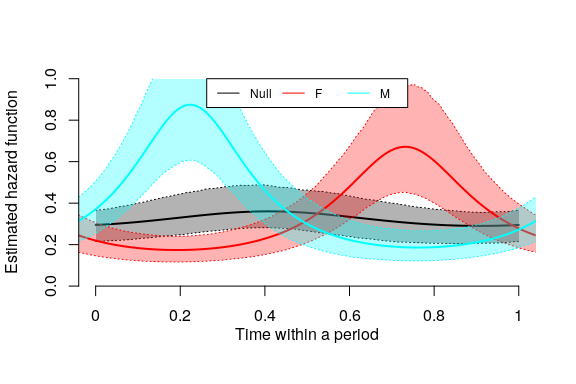## Data

Aside from the simulated sex-difference process, the package includes two anonymized and randomized caribou mortality data sets. The code below replicates the analyses presented in Gurarie et al. (in review).

### Northwest Territory woodland caribou

The first is from woodland caribou (Rangifer tarandus caribou) collected throughout the Northwest Territories in Canada, loaded and plotted as follows:

data(nwt_morts)
require(ggplot2); require(magrittr); require(plyr)
ggplot(nwt_morts %>% arrange(start) %>% mutate(id = factor(id, levels = id)),
aes(x = start, y = id, col = status)) +
geom_errorbarh(aes(xmin = start, xmax = end))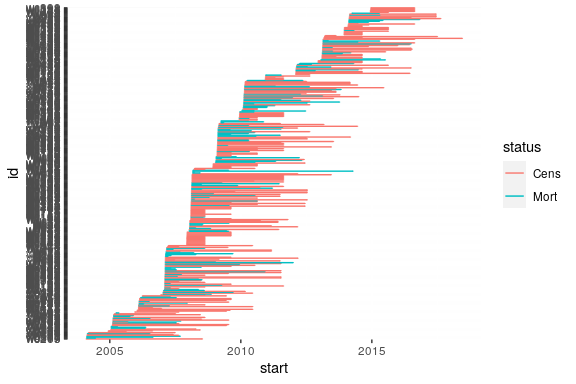Note these data have been randomized by year, such that the seasonal patterns are retained.

These data need to be converted to a cycloSurv data type, which inherits from the Surv class used in survival and other survival modeling packages:

nwt_surv = with(nwt_morts,
create_cycloSurv(start, end, event = status == "Mort",
period = 365, timeunit = "days"))

Note, it is important to specify the length of the period and time-unit.

Once these data are converted to the right class, they can be analyzed as in the examples above. For example, the Northwest Territories woodland caribou show three distinct seasons of higher mortality:

nwt_fit <- fit_cyclomort(nwt_surv, n.seasons = 3)
plot(nwt_fit, breaks = 40)
> Estimating 95% confidence intervals for the hazard function drawing 5000 samples from the parameter estimates.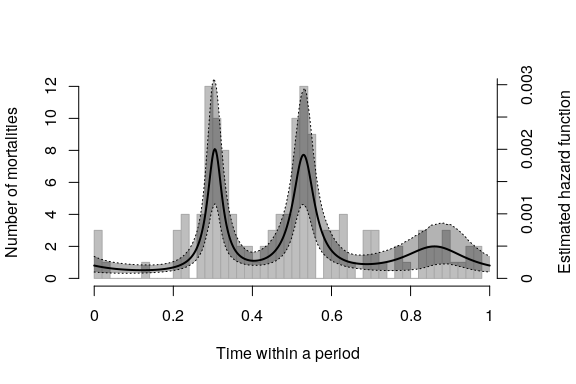summary(nwt_fit)
> $model >  "Multi-seasonal hazard function fit with 3 seasons with periodicity 365.\n\n" > >$estimates
> $estimates$meanhazard
>       estimate       CI.low      CI.high           se
> 1 0.0004687038 0.0004268173 0.0005105903 2.094325e-05
>
> $estimates$season 1
>   parameter season    estimate      CI.low     CI.high
> 1      peak      1 111.3347650 107.3628011 115.3067288
> 2  duration      1  16.6845474   9.4702941  28.6382052
> 3    weight      1   0.2935488   0.1834377   0.4036598
>
> $estimates$season 2
>   parameter season    estimate      CI.low     CI.high
> 1      peak      2 193.2154590 188.2757939 198.1551241
> 2  duration      2  24.3751539  12.8509741  43.8720870
> 3    weight      2   0.4126905   0.2422737   0.5831072
>
> $estimates$season 3
>   parameter season    estimate      CI.low     CI.high
> 1      peak      3 314.3449822 288.3571209 340.3328435
> 2  duration      3  67.8830410  24.5521768 127.1443242
> 3    weight      3   0.2937608   0.1185144   0.4690071
>
>
> $analysis >  "Log-likelihood: -324.6289; AIC: 667.2577" ### Western Arctic herd caribou The second data set is from the western Arctic herd (WAH) of migratory tundra caribou in western Alaska, U.S.: data(wah_morts) ggplot(wah_morts %>% arrange(start), aes(x = start, y = id, col = fate)) + geom_errorbarh(aes(xmin = start, xmax = end))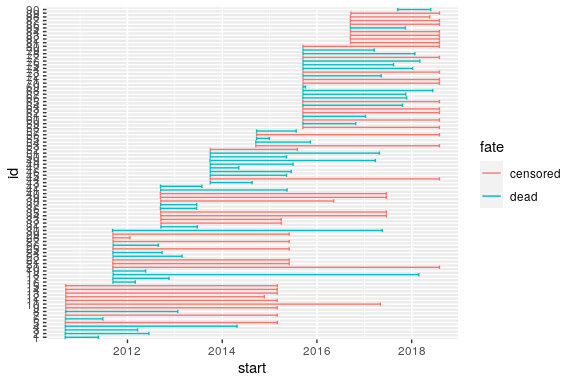The western Arctic herd, with two pronounced seasons of mortality, showed an increase in mortality in the 2017-2018 winter season, which we analyze by converting to a cycloSurv objects and censoring and trimming the data in September 2017 using two convenient helper functions, censor_cycloSurv and trim_cycloSurv: wah <- with(wah_morts, create_cycloSurv(start = start, end = end, event = fate == "dead", period = 365)) cutoff <- "2016-09-01" wah_pre = censor_cycloSurv(wah, censor.time = cutoff) wah_post = trim_cycloSurv(wah, trim.time = cutoff) wah_fit_pre <- fit_cyclomort(wah_pre, n.seasons = 1) > Warning in fit_cyclomort(wah_pre, n.seasons = 1): Upper confidence limit for > weights manually coerced to 1 wah_fit_post <- fit_cyclomort(wah_post, n.seasons = 1) > Warning in fit_cyclomort(wah_post, n.seasons = 1): Upper confidence limit for > weights manually coerced to 1 summary(wah_fit_pre) >$model
>  "Multi-seasonal hazard function fit with 1 seasons with periodicity 365.\n\n"
>
> $estimates >$estimates$meanhazard > estimate CI.low CI.high se > 1 0.000457814 0.0002812442 0.0006343837 8.828489e-05 > >$estimates$season 1 > parameter season estimate CI.low CI.high > 1 peak 1 163.22359 136.57761 189.8696 > 2 duration 1 82.45898 44.86029 123.4940 > > >$analysis
>  "Log-likelihood: -70.3382; AIC: 146.6764"
summary(wah_fit_post)
> $model >  "Multi-seasonal hazard function fit with 1 seasons with periodicity 365.\n\n" > >$estimates
> $estimates$meanhazard
>       estimate       CI.low    CI.high           se
> 1 0.0007734011 0.0004070717 0.00113973 0.0001831647
>
> $estimates$season 1
>   parameter season  estimate    CI.low  CI.high
> 1      peak      1  73.92278 -42.24560 190.0912
> 2  duration      1 143.30471  48.39557 177.3699
>
>
> \$analysis
>  "Log-likelihood: -40.0146; AIC: 86.0292"
plot(wah_fit_pre, hist= FALSE, ymax = 0.003, monthlabs = TRUE)
> Estimating 95% confidence intervals for the hazard function drawing 5000 samples from the parameter estimates.
plot(wah_fit_post, hist= FALSE, add = TRUE, hazcolor = "red")
> Estimating 95% confidence intervals for the hazard function drawing 5000 samples from the parameter estimates.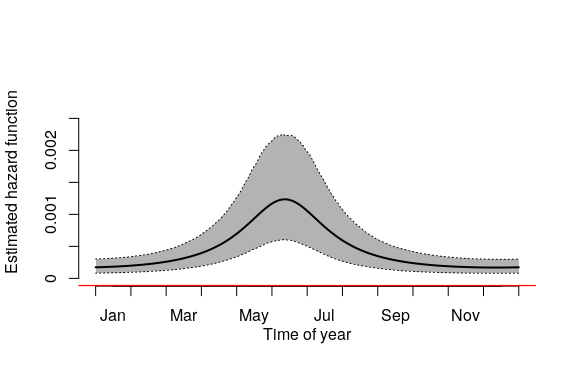Note that this result differs somewhat from that in Gurarie et al. (2020) since the data provided in the package are a subset of the complete data set, and too sparse to fit a more appropriate two season model.